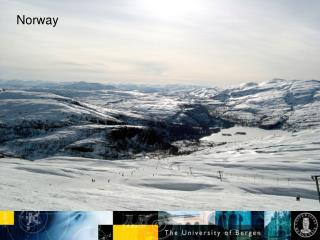DownloadDownload PresentationNorway

# Norway

Download Presentation## Norway

- - - - - - - - - - - - - - - - - - - - - - - - - - - E N D - - - - - - - - - - - - - - - - - - - - - - - - - - -
##### Presentation Transcript

1. Norway

2. 3-dim. QGP Fluid Dynamics and Flow Observables László Csernai (Bergen Computational Physics Lab., Univ. of Bergen)

3. Introduction • Strong flow is observed => • - Early, local eq., - EoS • nq scaling – QGP flows • no flow in hadronic matter > simultaneous hadronization and FO (HBT, high strangeness abundance)

4. Relativistic Fluid Dynamics Eg.: from kinetic theory. BTE for the evolution of phase-space distribution: Then using microscopic conservation laws in the collision integral C: These conservation laws are valid for any, eq. or non-eq. distribution, f(x,p). These cannot be solved, more info is needed! Boltzmann H-theorem: (i) for arbitrary f, the entropy increases, (ii) for stationary, eq. solution the entropy is maximal,   EoS P = P (e,n) Solvable for local equilibrium!

5. Relativistic Fluid Dynamics For any EoS, P=P(e,n), and any energy-momentum tensor in LE(!): Not only for high v!

6. Two theoretical problems • Initial state – • - Fitted initial states > moderate insight • Final Freeze Out • - Realistic Model, Continuos FO, ST layer, Non-eq. distribution

7. Stages of a Collision Freeze Out >>> Detectors Hadronization, chemical FO, kinetic FO -------------- One fluid >>> E O S Fluid components, Friction Local Equilibration, Fluids Collective flow reveals the EoS ifwe have dominantly one fluid with local equilibrium in a substantial part of the space-time domain of the collision !!!

8. Heavy Colliding System Initial state Idealizations FO Layer FO HS time QGP EoS One fluid Hadronization Chemical Freeze Out Kinetic Freeze Out

9. Fire streak picture - Only in 3 dimensions! Myers, Gosset, Kapusta, Westfall

10. String rope --- Flux tube --- Coherent YM field

11. Initial state 3rd flow component

12. 3-Dim Hydro for RHIC (PIC)

13. 3-dim Hydro for RHIC Energies Au+Au ECM=65 GeV/nucl. b=0.5 bmax Aσ=0.08 => σ~10 GeV/fm e [ GeV / fm3 ] T [ MeV] . . t=0.0 fm/c, Tmax= 420 MeV, emax= 20.0 GeV/fm3, Lx,y= 1.45 fm, Lz=0.145 fm 8.7 x 4.4 fm EoS: p= e/3 - B/3, B = 397 MeV/fm3

14. Au+Au ECM=65 GeV/nucl. b=0.5 bmax Aσ=0.08 => σ~10 GeV/fm e [ GeV / fm3 ] T [ MeV] . . t=9.1 fm/c, Tmax= 417 MeV, emax= 19.6 GeV/fm3, Lx,y= 1.45 fm, Lz=0.145 fm 20.3 x 5.8 fm

15. Au+Au ECM=65 GeV/nucl. b=0.5 bmax Aσ=0.08 => σ~10 GeV/fm e [ GeV / fm3 ] T [ MeV] . . t=18.2 fm/c, Tmax= 417 MeV, emax= 19.4 GeV/fm3, Lx,y= 1.45 fm, Lz=0.145 fm 34.8 x 8.7 fm

16. Directed Transverse flow Global Flow patterns: 3rd flow component (anti - flow) X Z b Squeeze out + Spherical flow Elliptic flow

17. 3rd flow component Csernai & Röhrich [Phys.Lett.B458(99)454] Hydro [Csernai, HIPAGS’93]

18. Preliminary “Wiggle”, Pb+Pb, Elab=40 and 158GeV [NA49] A. Wetzler v1< 0 158 GeV/A The “wiggle” is there!

19. Flow is a diagnostic tool Impact par. Equilibrationtime Transparency – string tension Consequence:v1(y), v2(y), …

20. FOHS - Movies: Freeze Out B=0, T-fo = 139MeV B=0.4, T-fo = 139MeV B=0, T-fo = 180MeV B=0.4, T-fo = 180MeV [Bernd Schlei, Los Alamos, LA-UR-03-3410]

21. (B) - Freeze out over FOHS- post FO distribution?= 1st.: n, T, u, cons. Laws != 2nd.: non eq. f(x,p) !!! ->(C) • (Ci) Simple kinetic model • (Cii) Covariant, kinetic F.O. description • (Ciii) Freeze out form transport equation • Note: ABC together is too involved!B & C can be done separately -> f(x,p)

22. Freeze out is : Stronglydirected process: Delocalized: Them.f.p. - reaches infinity Finite characteristic length Modified Boltzmann Transport Equation for Freeze Out description The Boltzmann Transport Equation and Freeze Out The change is not negligible in the FO direction

23. The invariant “ Escape” probability in finite layer The escape form theint to free component • Not to collide, depends on remaining distance • If the particle momentum is not normal to the surface, the spatial distance increases Early models: 1

24. The invariant “ Escape” probability A B C t’ x’ D E F [RFG] Escape probability factors for different points on FO hypersurface, in the RFG. Momentum values are in units of [mc]

25. Results – the cooling and retracting of the interacting matter [RFF] [RFF] Space-Like FO Time-Like FO cooling retracting  Cut-off factorflowvelocityNo Cut-off

26. [RFF] [RFF] Results – the contour lines of the FO distribution, f(p) Space-Like FO Time-Like FO jump in [RFF] With different initial flow velocities

27. Recent open, flow related issues • Is QGP a “perfect fluid” ? – • Small (?) viscosity, but strong interaction (?)- Laminar flow, not turbulent -> large viscosity- Cascades need high cross section to reproduce flow • Comprehensive flow assessment • - v1, v2, v3 … should be evaluated on equal footing - There is one reaction plane, , (not 123 … ) - y, , pT correlations are equally important (y ?) • Solution: Event by Event flow evaluation